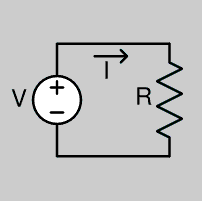## Ohms LawA voltage source, V, drives an electric current, I , through resistor, R, the three quantities obeying Ohm's law: V = IR

Ohm's law, named after its discoverer Georg Ohm , states that the potential difference between two points along a connected path and the current flowing through it are proportional at a given (i.e., fixed) temperature:

### V = I x R

Where V is the potential difference, I is the current, and R is a constant called the electrical resistance of the conductor.

The potential difference is the voltage drop from one point to a second point within the connected path of a conductor, and is sometimes designated by E or U instead of V. The unit of resistance is the ohm, which is equal to one volt per ampere, or one volt-second per coulomb.

The inverse of resistance, 1/R, is conductance, and its SI unit is the siemens (also unofficially called the mho). Conductance, or its alternating current (and frequency-dependent) analog admittance, is widely used in certain types of electrical and electronic analysis.

Ohms Law Calculator